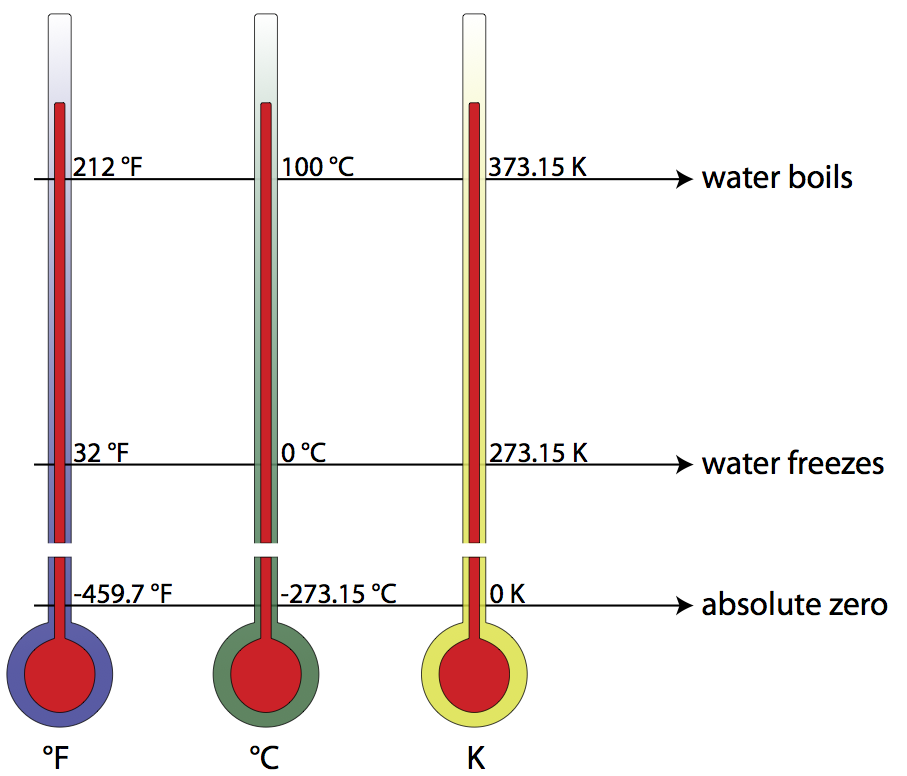# Temperature

In solids, the particles comprising the solid are held together tightly, therefore their motion is limited to vibrating back and forth in their given positions. In liquids, the particles can move back and forth across each other, but the object itself has no defined shape. In gases, the particles move throughout the volume available, interacting with each other and the walls of any container holding them. In all cases, the total thermal energy of the object is the sum total of the kinetic energies of its constituent particles.

Instead of just looking at the sum of all the individual particles’ kinetic energies, you could examine the average kinetic energy of the particles comprising the object, realizing that the actual kinetic energies of individual particles may vary significantly. The average kinetic energy of the particles is directly related to the temperature of the object by the following equation:Examining this equation, the average kinetic energy is given in Joules, kB is Boltzmann’s Constant (1.38×10-23 J/K), and the temperature is given in Kelvins, the SI unit of temperature. Note that even though two objects can have the same temperature (and therefore the same average kinetic energy), they may have different internal energies.The Kelvin scale is closely related to the Celsius temperature scale, but where the Celsius scale targets the freezing point of water as 0° C, the Kelvin scale utilizes its zero at what is known as absolute zero (the point on a Volume vs. Temperature graph for a gas where the extended curve would hypothetically reach zero volume), considered a theoretical minimum temperature. There- fore, absolute zero is 0 Kelvins, which is equivalent to -273.15° Celsius. To convert from Kelvins to degrees Celsius, just add 273.15 to your temperature reading in degrees Celsius. The freezing point of water, then, is 0°C or 273.15 K, and the boiling point of water is 100°C or 373.15 K. Compare this to the Fahrenheit scale, where water freezes at 32°F, and boils at 212°F!Question: What is the average kinetic energy of the molecules in a steak at a temperature of 345 Kelvins?Answer:Question: Normal canine body temperature is 101.5°F. What is normal canine body temperature in degrees Celsius? In Kelvins?Answer:Question: Given that the average kinetic energy of the particles comprising our sun is 1.2×10-19 J, find the temperature of the sun.

Answer:Question:Which graph best represents the relationship between the average kinetic energy (Kavg) of the random motion of the molecules of an ideal gas and its absolute temperature (T)?Answer: (2) since Kavg is a linear function of absolute temperature T.

Question: While orbiting Earth, the space shuttle has recorded temperatures ranging from 398K to 118K. These temperatures correspond to Celsius temperatures ranging from

1. 125°C to -391°C
2. 125°C to -155°C
3. 671°C to 391°C
4. 671°C to 155°C

Question:The temperature at which no thermal energy can be transferred from one object to another is

1. -273 K
2. 0 K
3. 0°C
4. 273°C

Answer: (2) 0 K is absolute zero.